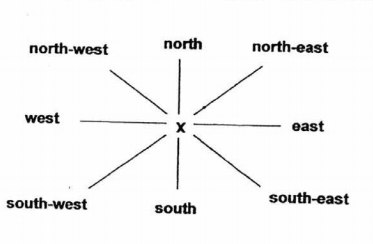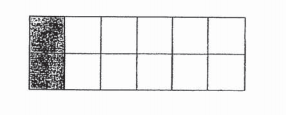# Primary 4 CA1 Practice #4

Time elapsed:
Q1. Round 29 105 to the nearest ten.     [1 mark]
Q2. What must be added to 712 to get 56 ?     [1 mark]
Q3. When a number is divided by 6, the quotient is 244 and the remainder is 5. What is the number?     [1 mark]
Q4. William is at point X facing north-west. He makes a 90° clockwise turn. Then he makes a _____ in the anti-clockwise direction to face south-east.     [1 mark]Q5. The digit 3 in one of the following numbers has the greatest value. Which number is it?     [2 marks]
Q6. What is the sum of the common factors of 16 and 24?     [1 mark]
Q7. The number of audience at a concert is 130 when rounded off to the nearest 10. What is the smallest possible number of audience at the concert?     [1 mark]
Q8. What is the sum of all the common factors of 15 and 30?     [1 mark]
Q9. 1304 x 7 = ____.     [1 mark]
Q10. Lucy wanted to shade 34 of the figure below. How many more squares must she shade?     [1 mark]Q11. When a number is divided by 6, the quotient is 7 and the remainder is 4. What is the number?     [2 marks]
Q12. Which of the following has the greatest value?     [1 mark]
Q13. Find the product of 134 and 35.     [1 mark]
Q14. What is the quotient when 923 is divided by 5?     [1 mark]
Q15. Lucy packs 18 boxes of egg tarts. Each box contains 24 egg tarts. If there are 15 egg tarts left unpacked, how many egg tarts are there altogether?     [1 mark]
Q16. When 6754 is divided by 7, what is the remainder?     [1 mark]
Q17. There are 110 rows of seats in an auditorium. Each row has 32 seats. 47 seats are occupied. How many seats are not occupied?     [1 mark]
Q18. Zoe walks 1545 m to school every day. What is the total distance she walks in 5 days? Round off your answer to the nearest hundred?     [1 mark]
Q19. What is the remainder when 1928 is divided by 6?     [1 mark]
Q20. Which of the following sets of numbers is arranged from the greatest to the smallest?     [1 mark]